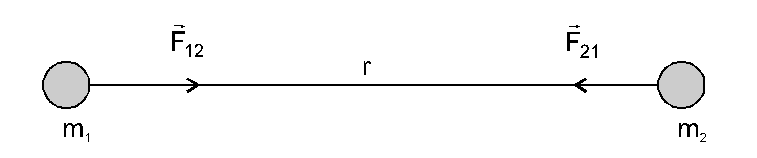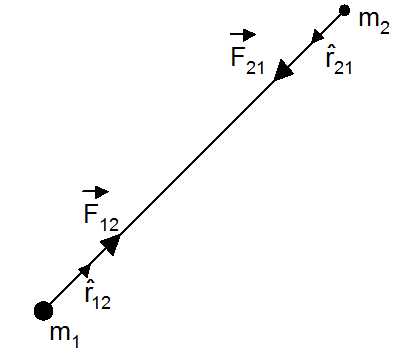Most Affordable JEE | NEET | 8,9,10 Preparation by Kota's Top IITian Doctor Faculties

# Universal law of gravitation class 11 - Physics, Gravitation - eSaral`
Hey, are you in Class 11? or do you want to learn about the universal law of gravitation for class 11? If yes. Then you are at the right place.

## Newton's Universal Law of Gravitation

Any two particles of matter anywhere in the universe attract each other with a force which is directly proportional to the product of their masses and inversely proportional to the square of the distance between them, the direction of the force being along the line joining the particles, i.e.

$\mathrm{F} \propto \frac{\mathrm{m}_{1} \mathrm{~m}_{2}}{\mathrm{r}^{2}}$

Or

$F=\frac{G m_{1} m_{2}}{r^{2}}$

G is the universal gravitational constant.

It does not depend on any physical factor and intervening medium.

If

$m_{1}=m_{2}=1 k g$,

$r=1 \mathrm{~m}$ then $\mathrm{F}=\mathrm{G}$

The gravitational constant is defined as the force of attraction acting between two-unit masses placed at a unit distance apart. G is a scalar quantity.

$G=6.673 \times 10^{-11} \mathrm{Nm}^{2} / \mathrm{kg}^{2}$

Gravitational forces are always attractive.
1. Gravitational force always acts along the line joining the two masses2. Gravitational force is a mutual force hence it is action-reaction force, i.e., $\vec{F}_{12}=-\vec{F}_{21}$

3. The value of G is small therefore gravitational force is weaker than electrostatic and nuclear forces.
4. Gravitational forces are conservative forces.

5. Gravitational forces is a central force because $F \propto \frac{1}{r^{2}}$.

6. The gravitational force between two masses is independent of the presence of other objects and the medium between the two masses.

7. Vector form of gravitational force is$\overrightarrow{\mathrm{F}}_{21}=-\frac{\mathrm{Gm}_{1} \mathrm{~m}_{2}}{\mathrm{r}_{12}^{2}} \hat{\mathrm{r}}_{12}$

force on $\mathrm{m}_{2}$ due to $\mathrm{m}_{1}$.

$\overrightarrow{\mathrm{F}}_{12}=-\frac{\mathrm{Gm}_{1} \mathrm{~m}_{2}}{\mathrm{r}_{21}^{2}} \hat{\mathrm{r}}_{21}$

force on $\mathrm{m}_{1}$ due to $\mathrm{m}_{2}$.

8. Dimensional formula of $\mathrm{G}$ is $\left[\mathrm{M}^{-1} \mathrm{~L}^{3} \mathrm{~T}^{-2}\right]$

9. Range is very large up to interstellar separation.

So, that's all from this Topic. I hope you get the idea about the universal law of gravitation for class 11. If you enjoyed this explanation then please share it with your friends.